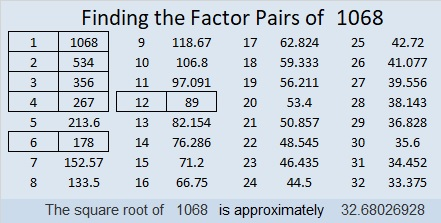# 1068 and Level 6

The permissible common factors of 40 and 48 are 4 and 8, and the permissible common factors of 54 and 36 are 6 and 9. By themselves, neither of those pairs of clues is enough to get you started with this level 6 puzzle. You will have to study ALL the clues to begin to solve it. Believe it or not, sometimes a row or column with no clue is the best place to start.Print the puzzles or type the solution in this excel file: 12 factors 1063-1072

This is my 1068th post so let me share a little information about the number 1068:

• 1068 is a composite number.
• Prime factorization: 1068 = 2 × 2 × 3 × 89, which can be written 1068 = 2² × 3 × 89
• The exponents in the prime factorization are 2, 1, and 1. Adding one to each and multiplying we get (2 + 1)(1 + 1)(1 + 1) = 3 × 2 × 2 = 12. Therefore 1068 has exactly 12 factors.
• Factors of 1068: 1, 2, 3, 4, 6, 12, 89, 178, 267, 356, 534, 1068
• Factor pairs: 1068 = 1 × 1068, 2 × 534, 3 × 356, 4 × 267, 6 × 178, or 12 × 89,
• Taking the factor pair with the largest square number factor, we get √1068 = (√4)(√267) = 2√267 ≈ 32.680271068 is the hypotenuse of a Pythagorean triple:
468-960-1068 which is 12 times (39-80-89)

This site uses Akismet to reduce spam. Learn how your comment data is processed.